The OpenFOAM FoundationSymmTensor2D< Cmpt > Class Template Reference

Templated 2D symmetric tensor derived from VectorSpace adding construction from 4 components, element access using xx(), xy() etc. member functions and the inner-product (dot-product) and outer-product of two Vectors (tensor-product) operators. More...

Inheritance diagram for SymmTensor2D< Cmpt >: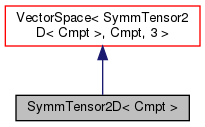[legend]
Collaboration diagram for SymmTensor2D< Cmpt >: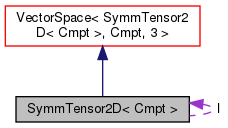[legend]

## Public Types

enum  components { XX, XY, YY }
Component labeling enumeration. More...

typedef SymmTensor2D< labellabelType
Equivalent type of labels used for valid component indexing. More...Public Types inherited from VectorSpace< SymmTensor2D< Cmpt >, Cmpt, 3 >
typedef VectorSpace< SymmTensor2D< Cmpt >, Cmpt, Ncmpts > vsType
VectorSpace type. More...

typedef Cmpt cmptType
Component type. More...

## Public Member Functions

SymmTensor2D ()
Construct null. More...

SymmTensor2D (const Foam::zero)
Construct initialized to zero. More...

SymmTensor2D (const VectorSpace< SymmTensor2D< Cmpt >, Cmpt, 3 > &)
Construct given VectorSpace. More...

SymmTensor2D (const SphericalTensor2D< Cmpt > &)
Construct given SphericalTensor. More...

SymmTensor2D (const Cmpt txx, const Cmpt txy, const Cmpt tyy)
Construct given the three components. More...

SymmTensor2D (Istream &)
Construct from Istream. More...

const Cmpt & xx () const

const Cmpt & xy () const

const Cmpt & yy () const

Cmpt & xx ()

Cmpt & xy ()

Cmpt & yy ()

const SymmTensor2D< Cmpt > & T () const
Transpose. More...

void operator= (const SphericalTensor2D< Cmpt > &)
Construct given SphericalTensor2D. More...Public Member Functions inherited from VectorSpace< SymmTensor2D< Cmpt >, Cmpt, 3 >
VectorSpace ()
Construct null. More...

VectorSpace (const Foam::zero)
Construct initialized to zero. More...

VectorSpace (Istream &)
Construct from Istream. More...

VectorSpace (const VectorSpace< Form2, Cmpt2, Ncmpts > &)
Construct as copy of a VectorSpace with the same size. More...

const Cmpt & component (const direction) const

Cmpt & component (const direction)

void component (Cmpt &, const direction) const

void replace (const direction, const Cmpt &)

const ConstBlock< SubVector, BStart > block () const

const VectorSpace< SymmTensor2D< Cmpt >, Cmpt, Ncmpts >::template ConstBlock< SubVector, BStart > block () const

const Cmpt & operator[] (const direction) const

Cmpt & operator[] (const direction)

void operator+= (const VectorSpace< SymmTensor2D< Cmpt >, Cmpt, Ncmpts > &)

void operator-= (const VectorSpace< SymmTensor2D< Cmpt >, Cmpt, Ncmpts > &)

void operator= (const Foam::zero)

void operator*= (const scalar)

void operator/= (const scalar)

## Static Public Attributes

static const direction rank = 2
Rank of SymmTensor2D is 2. More...

static const SymmTensor2D IStatic Public Attributes inherited from VectorSpace< SymmTensor2D< Cmpt >, Cmpt, 3 >
static const direction dim
Dimensionality of space. More...

static const direction nComponents
Number of components in this vector space. More...

static const direction mRows

static const direction nCols

static const char *const typeName

static const char *const componentNames []

static const SymmTensor2D< Cmpt > zero

static const SymmTensor2D< Cmpt > one

static const SymmTensor2D< Cmpt > max

static const SymmTensor2D< Cmpt > min

static const SymmTensor2D< Cmpt > rootMax

static const SymmTensor2D< Cmpt > rootMinStatic Public Member Functions inherited from VectorSpace< SymmTensor2D< Cmpt >, Cmpt, 3 >
static direction size ()
Return the number of elements in the VectorSpace = Ncmpts. More...

static SymmTensor2D< Cmpt > uniform (const Cmpt &s)
Return a VectorSpace with all elements = s. More...Public Attributes inherited from VectorSpace< SymmTensor2D< Cmpt >, Cmpt, 3 >
Cmpt v_ [Ncmpts]
The components of this vector space. More...

## Detailed Description

### template<class Cmpt> class Foam::SymmTensor2D< Cmpt >

Templated 2D symmetric tensor derived from VectorSpace adding construction from 4 components, element access using xx(), xy() etc. member functions and the inner-product (dot-product) and outer-product of two Vectors (tensor-product) operators.

Source files

Definition at line 53 of file SymmTensor2D.H.

## ◆ labelType

 typedef SymmTensor2D

Equivalent type of labels used for valid component indexing.

Definition at line 61 of file SymmTensor2D.H.

## ◆ components

 enum components

Component labeling enumeration.

Enumerator
XX
XY
YY

Definition at line 76 of file SymmTensor2D.H.

## ◆ SymmTensor2D() [1/6]

 SymmTensor2D ( )
inline

Construct null.

Definition at line 32 of file SymmTensor2DI.H.

Referenced by SymmTensor2D< Cmpt >::SymmTensor2D().

Here is the caller graph for this function: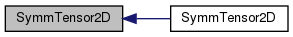## ◆ SymmTensor2D() [2/6]

 SymmTensor2D ( const Foam::zero )
inline

Construct initialized to zero.

Definition at line 37 of file SymmTensor2DI.H.

References SymmTensor2D< Cmpt >::SymmTensor2D().

Here is the call graph for this function: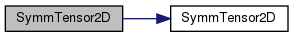## ◆ SymmTensor2D() [3/6]

 SymmTensor2D ( const VectorSpace< SymmTensor2D< Cmpt >, Cmpt, 3 > & vs )
inline

Construct given VectorSpace.

Definition at line 45 of file SymmTensor2DI.H.

## ◆ SymmTensor2D() [4/6]

 SymmTensor2D ( const SphericalTensor2D< Cmpt > & st )
inline

Construct given SphericalTensor.

Definition at line 54 of file SymmTensor2DI.H.

Here is the call graph for this function: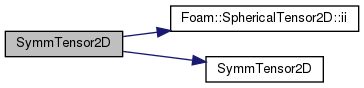## ◆ SymmTensor2D() [5/6]

 SymmTensor2D ( const Cmpt txx, const Cmpt txy, const Cmpt tyy )
inline

Construct given the three components.

Definition at line 63 of file SymmTensor2DI.H.

## ◆ SymmTensor2D() [6/6]

 SymmTensor2D ( Istream & is )
inline

Construct from Istream.

Definition at line 74 of file SymmTensor2DI.H.

## ◆ xx() [1/2]

 const Cmpt & xx ( ) const
inline

Definition at line 83 of file SymmTensor2DI.H.

Here is the caller graph for this function: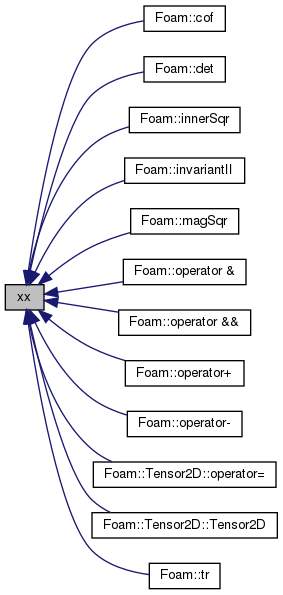## ◆ xy() [1/2]

 const Cmpt & xy ( ) const
inline

Definition at line 89 of file SymmTensor2DI.H.

Here is the caller graph for this function: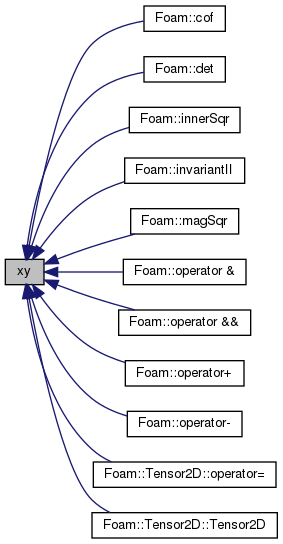## ◆ yy() [1/2]

 const Cmpt & yy ( ) const
inline

Definition at line 95 of file SymmTensor2DI.H.

Here is the caller graph for this function: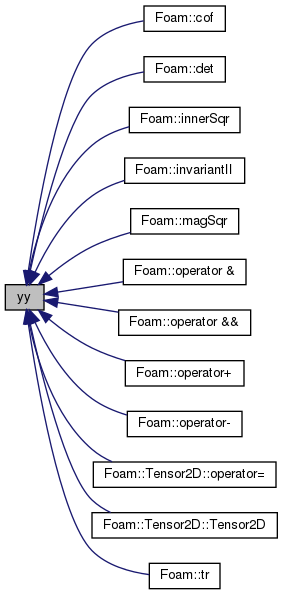## ◆ xx() [2/2]

 Cmpt & xx ( )
inline

Definition at line 102 of file SymmTensor2DI.H.

## ◆ xy() [2/2]

 Cmpt & xy ( )
inline

Definition at line 108 of file SymmTensor2DI.H.

## ◆ yy() [2/2]

 Cmpt & yy ( )
inline

Definition at line 114 of file SymmTensor2DI.H.

## ◆ T()

 const Foam::SymmTensor2D< Cmpt > & T ( ) const
inline

Transpose.

Definition at line 121 of file SymmTensor2DI.H.

## ◆ operator=()

 void operator= ( const SphericalTensor2D< Cmpt > & st )
inline

Construct given SphericalTensor2D.

Definition at line 131 of file SymmTensor2DI.H.

## ◆ rank

 const direction rank = 2
static

Rank of SymmTensor2D is 2.

Definition at line 67 of file SymmTensor2D.H.

## ◆ I

 const Foam::symmTensor2D I
static

Definition at line 72 of file SymmTensor2D.H.

The documentation for this class was generated from the following files: